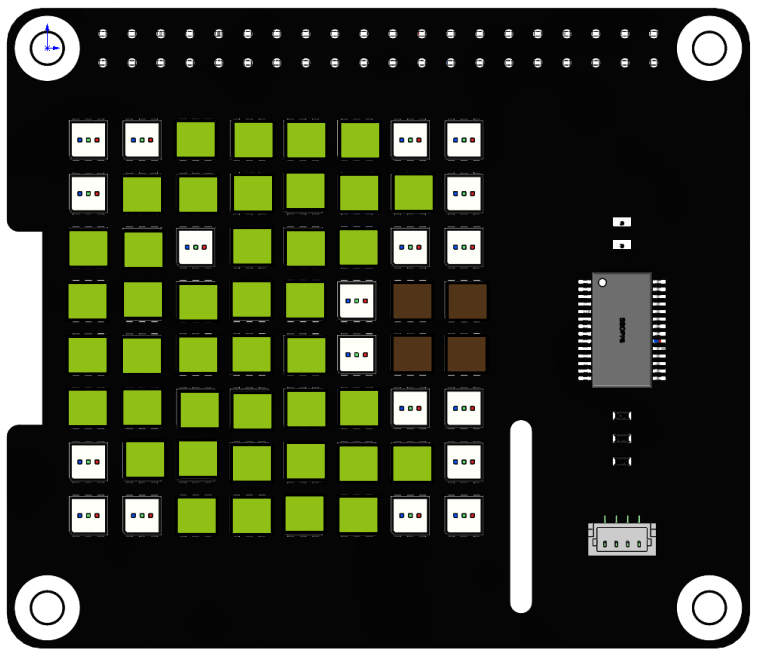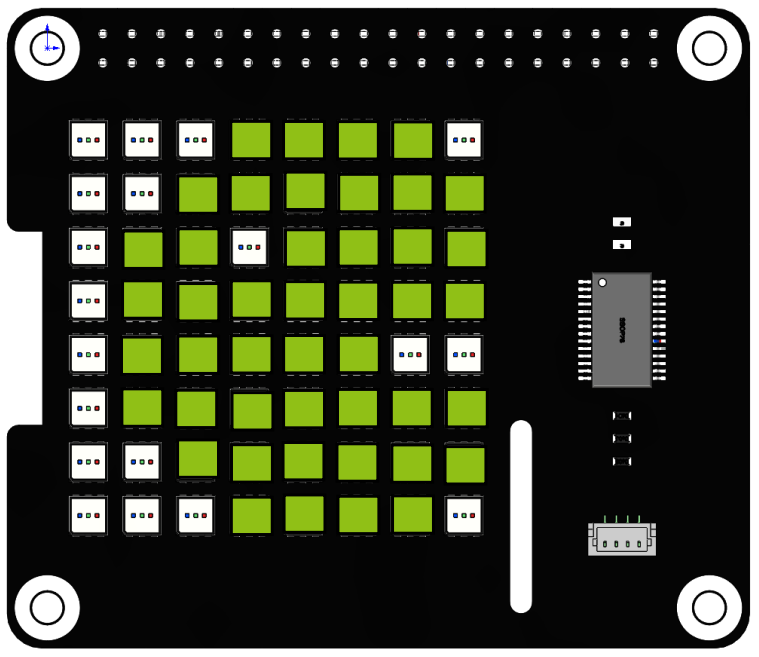# Custom Dynamic Shape¶

Here, based on the previous project, a continuous animation of Pac-Man eating dots will be created.Run the code

When the program is running, you will see Pac-Man on the RGB matrix cap move from left to right and leave after eating the rightmost dot.

```cd /home/pi/rgb_matrix/raspberrypi
sudo python3 custom_dynamic_shape.py
```

Code

Note

You can Modify/Reset/Copy/Run/Stop the code below. But before that, you need to go to source code path like `rgb_matrix/raspberrypi`. After modifying the code, you can run it directly to see the effect.

```from rgb_matrix import RGB_Matrix
import time
import random

def pacman(a,k):

list2 = [[a-4,0,a-1,0],
[a-5,1,a,1],
[a-6,2,a-5,2],
[a-3,2,a-1,2],
[a-6,3,a-2,3],
[a-6,4,a-2,4],
[a-6,5,a-1,5],
[a-5,6,a,6],
[a-4,7,a-1,7]]

fill = (144,192,22)
for i in range(0,k+1):
for j in list2:
rr.draw_line(j,fill)

rr.display()

for j in list2:
rr.draw_line(j,fill=(0,0,0))

for i in range(0,9):
list2[i] += 1
list2[i] += 1

time.sleep(0.1)

def pacman2():

rr.draw_rectangle(rectangle_coor,fill=(0,0,0))

list = [[2,0,5,0],
[1,1,6,1],
[0,2,1,2],
[3,2,7,2],
[0,3,7,3],
[0,4,3,4],
[0,5,7,5],
[1,6,6,6],
[2,7,5,7]]

fill = (144,192,22)
for i in list:
rr.draw_line(i,fill)

rr.display()
time.sleep(0.1)

rr.draw_rectangle(rectangle_coor,fill=(0,0,0))

def pac():

coor = [6,3,7,4]
rr.draw_rectangle(coor,fill=(82,52,25))

if __name__ == "__main__":
rr = RGB_Matrix(0X74)

rectangle_coor = [0,0,7,7]

while True:
pac()
pacman(0,6)
pacman2()
pacman(6,7)
```

How it works?

```while True:
pac()
pacman(0,6)
pacman2()
pacman(6,7)
```

We can disassemble Pac-Man into three actions, `pac()` represents the position of the dot.

• `pacman(0,6)` means that Pac-Man moves from the far left to the side of the dot.

• `pacman2()` mouth closed to indicate the action of eating.

• `pacman(6,7)` indicates to continue to leave after eating.

```def pacman(a,k):

list2 = [[a-4,0,a-1,0],
[a-5,1,a,1],
[a-6,2,a-5,2],
[a-3,2,a-1,2],
[a-6,3,a-2,3],
[a-6,4,a-2,4],
[a-6,5,a-1,5],
[a-5,6,a,6],
[a-4,7,a-1,7]]

fill = (144,192,22)
for i in range(0,k+1):
for j in list2:
rr.draw_line(j,fill)

rr.display()

for j in list2:
rr.draw_line(j,fill=(0,0,0))

for i in range(0,9):
list2[i] += 1
list2[i] += 1

time.sleep(0.1)
```

The `pacman()` function is used to make Pac-man move from the left to the right in an open-mouthed state until it disappears. It has two parameters `a` and `k`, `a` represents the starting position of Pac-man and `k` represents the number of squares moved to the right.

• `list2` stores the coordinates of Pac-man’s open-mouth state, drawn as lines, with the x-coordinate of each line determined by `a`.

• Define a two-level for loop. The inner loop does three things: draws Pac-Man, moves each line in `list2` one square to the right, and removes the movement.

• The outer layer repeats the loop `k` times, which means Pac-Man moves `k` squares to the right.